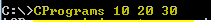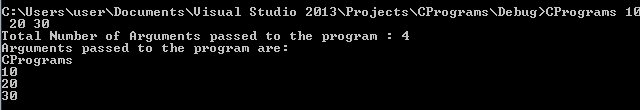# Command Line Arguments in C Programming

## Command Line Arguments

Any program is composed of inputs and outputs. Inputs are given to the program by the user using keyboard, files or command lines. We have already seen how to insert values from keyboard (scanf, getchar, gets) and files (fscanf, fgetc, fgets). C provides another method to input the values to the program using command line. In this method, input values are passed while executing the program itself. Every time the program is executed, we have to pass the arguments as input values and it can be different each time depending on the requirement.Here Cprograms is the executable program name that needs to be executed, (10, 20, 30) are the input values to the program passed while executing the program. It need not be integer value, it can be of any datatype.

These input values are captured by the parameters of the main () function. When we pass the input values using command line, we have to have input parameters to the main function like below:

```type main(int argc, char *argv[]);

int main(int argc, char *argv[]);```

where type is the datatype of the return value of the main function. The argument argc is the integer argument which contains the total number of the arguments passed via command line. In above example we have 4 arguments passed through command line. Even the executable program name is also considered as one of the arguments passed. Argument count starts from 0 to N-1 for N arguments. Next parameter is argv which is an array pointer to characters. It actually contains the list of arguments passed in the command line. In above example, argv = Cprograms, argv =10, argv = 20 and argv =30.

Below program shows the usage and value at argc and argv.

```#include

int main(int argc, char *argv[]){
printf("Total Number of Arguments passed to the program : %d", argc);

printf("\nArguments passed to the program are:\n");
for (int i = 0; i < argc; i++)
printf("%s\n", argv[i]);
}```Translate »